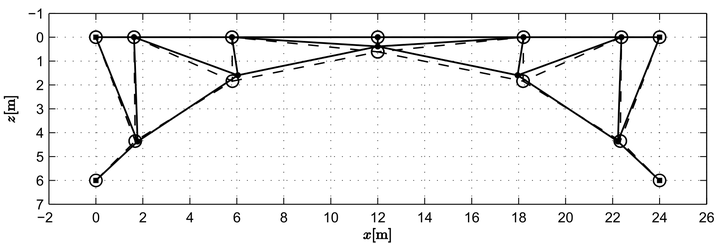# Structural optimization using the energy method with integral material behaviour### Abstract

With the advances of the computer technology, structural optimization has become a prominent field in structural engineering. In this study an unconventional approach of structural optimization is presented which utilize the Energy method with Integral Material behaviour (EIM), based on the Lagrange’s principle of minimum potential energy. The equilibrium condition with the EIM, as an alternative method for nonlinear analysis, is secured through minimization of the potential energy as an optimization problem. Imposing this problem as an additional constraint on a higher cost function of a structural property, a bilevel programming problem is formulated. The nested strategy of solution of the bilevel problem is used, treating the energy and the upper objective function as separate optimization problems. Utilizing the convexity of the potential energy, gradient based algorithms are employed for its minimization and the upper cost function is minimized using the gradient free algorithms, due to its unknown properties. Two practical examples are considered in order to prove the efficiency of the method. The first one presents a sizing problem of I steel section within encased composite cross section, utilizing the material nonlinearity. The second one is a discrete shape optimization of a steel truss bridge, which is compared to a previous study based on the Finite Element Method.

Type
Publication
In Proceedings of the 20th International Conference on the Application of Computer Science and Mathematics in Architecture and Civil Engineering, Weimar, Germany